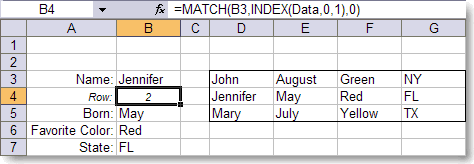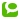Last week I talked about an Excel function called VLOOKUP. It's a nice enough function, but has 1 major flaw: it's pretty slow, and when you have to look-up several other column info for the same key, it gets much slower.
Using the MATCH function, combined with the INDEX function, you get a lot of power, and both of them are very fast.
I'm talking about the following scenario:Note the formula in cell B4.
MATCH has 3 arguments:

• The value to look for
• The 1 column (or row) range to look into
• The match-type: 0 means an exact match (but not case sensitive)
MATCH return the row (or column) number of the range where the value is found.

The range D2 to G5 is called 'Data'.
In order to get a range, consisting of the first column of the range, I use the INDEX function.
INDEX has 3 easy arguments:
• The range to look into
• The row number
• The column number
Supplying a 0 for the row number (as I did in the formula above), gives you all the rows of the specified column.
As the MATCH function reveals that Jennifer is the second in the list, we can now use the INDEX function again to retrieve the remaining data.
So cell B5 has the following formula:
=INDEX(Data,B4,2)
Returning the value of the second row, second column of the range 'Data'.
INDEX is extremely fast, and we only have to do 1 'look-up'.
If you need to do thousands of look-ups, you will see the difference !

Category:  Microsoft Excel  |Technorati: ,